# Check Out Correct Answers For yesterday WAEC MathematicS.

Today being the 17th of August 2020, the secondary school exit students finally sat for their first WAEC examination which happened to be Mathematics.

Below are the correct answers for today’s WAEC Exams:

(1a)

Given A={2,4,6,8,…}

B={3,6,9,12,…}

C={1,2,3,6}

U= {1,2,3,4,5,6,7,8,9,10}

A’ = {1,3,5,7,9}

B’ = {1,2,4,5,7,8,10}

C’ = {4,5,7,8,9,10}

A’nB’nC’ = {5, 7}

(1b)

Cost of each premiere ticket = \$18.50

At bulk purchase, cost of each = \$80.00/50 = \$16.00

Amount saved = \$18.50 – \$16.00

=\$2.50

-=-=-=-=-=-=

3a)

Draw the diagram

CBD = CDB(Base angles of an issoceles triangle)

BCD + CBD + CDB = 180°(sum of angles in a triangle)

2CDB + BCD = 180°

2CDB + 108° = 180°

2CDB = 180° – 108° =72°

CDB = 72/2 = 36°

BDE = 90°(angle in a semi-circle)

CDE = CDB + BDE

= 36° + 90°

= 126°

(3b)

(CosX)² – SinX/(SinX)²+ CosX

Using Pythagoras theorem, third side of triangle

y² = 1² + |3²

y² = 1 + 3 = 4

y = square root e = 2

Sin X = root 3/2(opp/hyp)

(CosX)² – SinX/(SinX)² + CosX

= (1/2)² – root3/2 / (root3/2)² + 1/2

= 1/4 – root3/2 / 3/4 + 1/2

= 1 – 2root3/4 / 3+2/4

= 1-2root3/5

-=-=-=-=

(8a)

Let Ms Maureen’s Income = Nx

1/4x = shopping mall

1/3x = at an open market

Hence shopping mall and open market = 1/4x + 1/3x

= 3x + 4x/12 = 7/12x

Hence the remaining amount

= X-7/12x = 12x-7x/12 =5x/12

Then 2/5(5x/12) = mechanic workshop

= 2x/12 = x/6

Amount left = N225,000

Total expenses

= 7/12x + X/6 + 225000

= Nx

7x+2x+2,700,000/12 =Nx

9x + 2,700,000 = 12x

2,700,000 = 12x – 9x

2,700,000/3 = 3x/3

X = N900,000

(ii) Amount spent on open market = 1/3X

= 1/3 × 900,000

= N300,000

(8b)

T3 = a + 2d = 4m – 2n

T9 = a + 8d = 2m – 8n

-6d = 4m – 2m – 2n + 8n

-6d = 2m + 6n

-6d/-6 = 2m+6n/-6

d = -m/3 – n

d = -1/3m – n

-=-=-=-=-=-=-=-=

(9a)

Draw the triangle

(9b)

(i)Using cosine formulae

q² = x² + y² – 2xycosQ

q² = 9² + 5² – 2×9×5cos90°

q² = 81 + 25 – 90 × 0

q² = 106

q = square root 106

q = 10.30 = 10km/h

Distance = 10 × 2 = 20km

(ii)

Using sine formula

y/sin Y = q/sin Q

5/sin Y = 10.30/sin 90°

Sin Y = 5 × sin90°/10.30

Sin Y = 5 × 1/10.30

Sin Y = 0.4854

Y = sin‐¹(0.4854), Y = 29.04

Bearing of cyclist X from y

= 90° + 19.96°

= 109.96° = 110°

(9c)

Speed = 20/4, average speed = 5km/h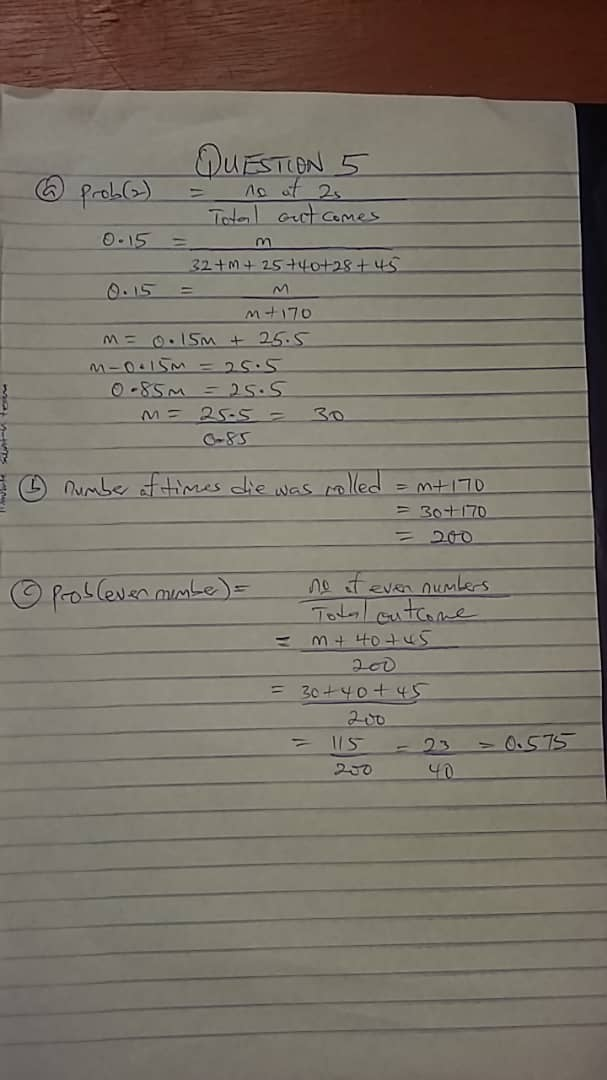View pictures in App save up to 80% data.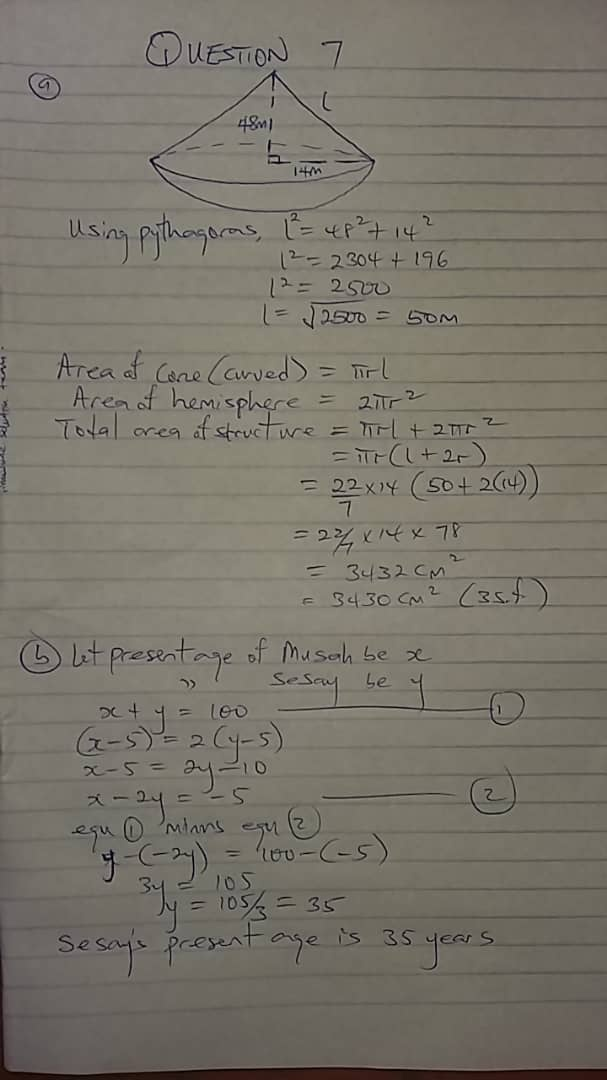View pictures in App save up to 80% data.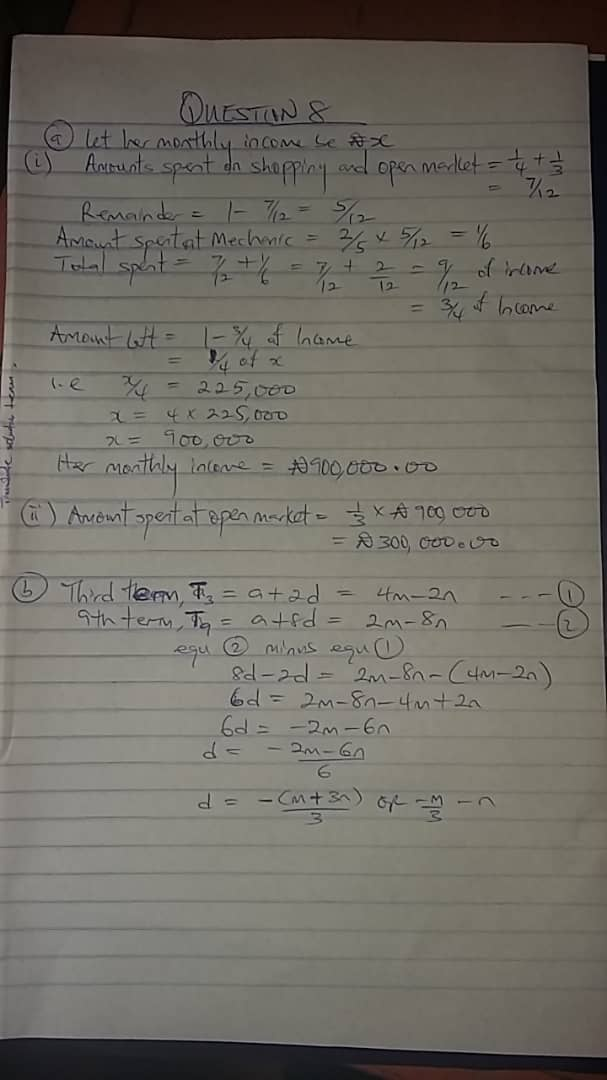View pictures in App save up to 80% data.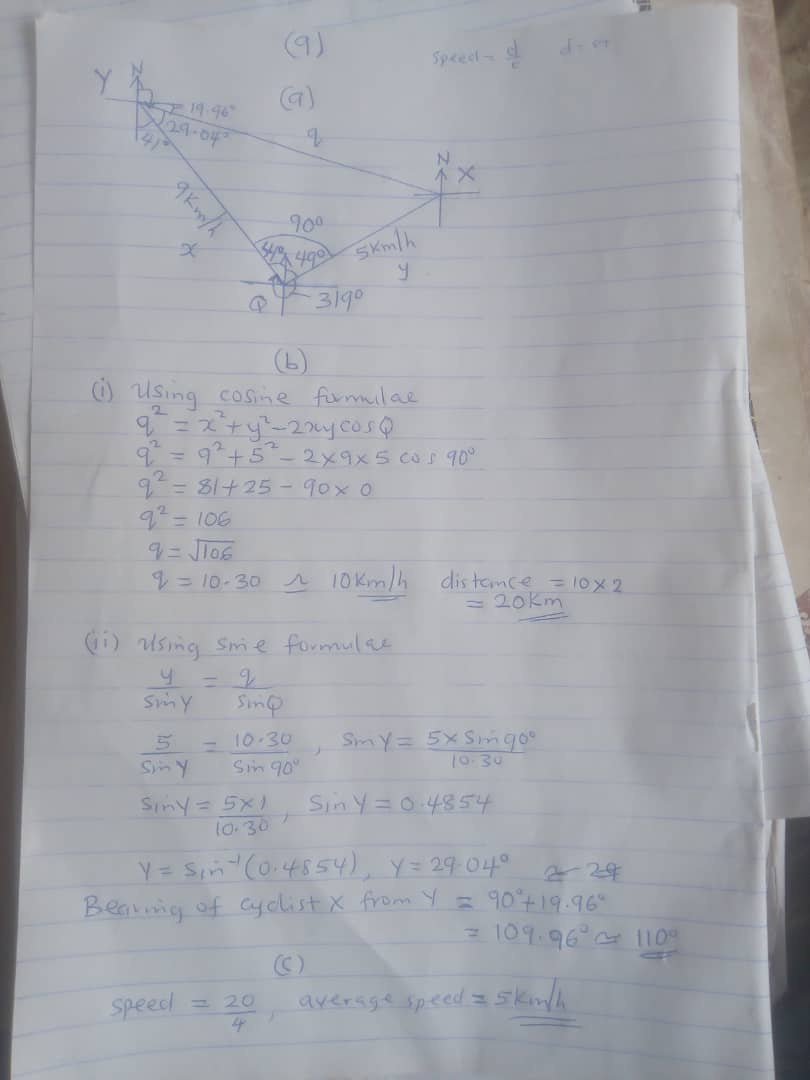View pictures in App save up to 80% data.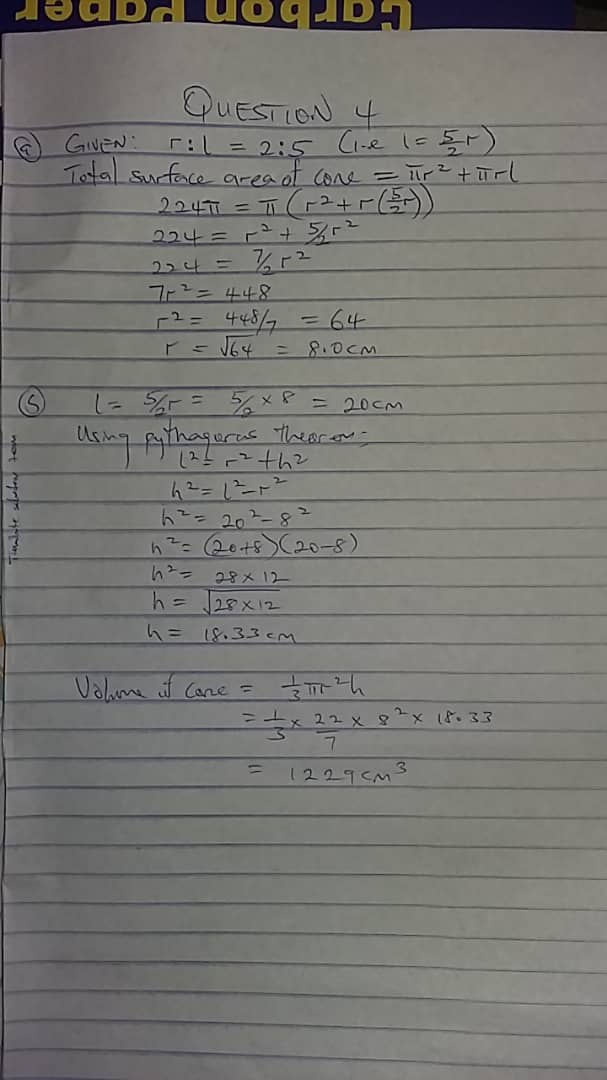View pictures in App save up to 80% data.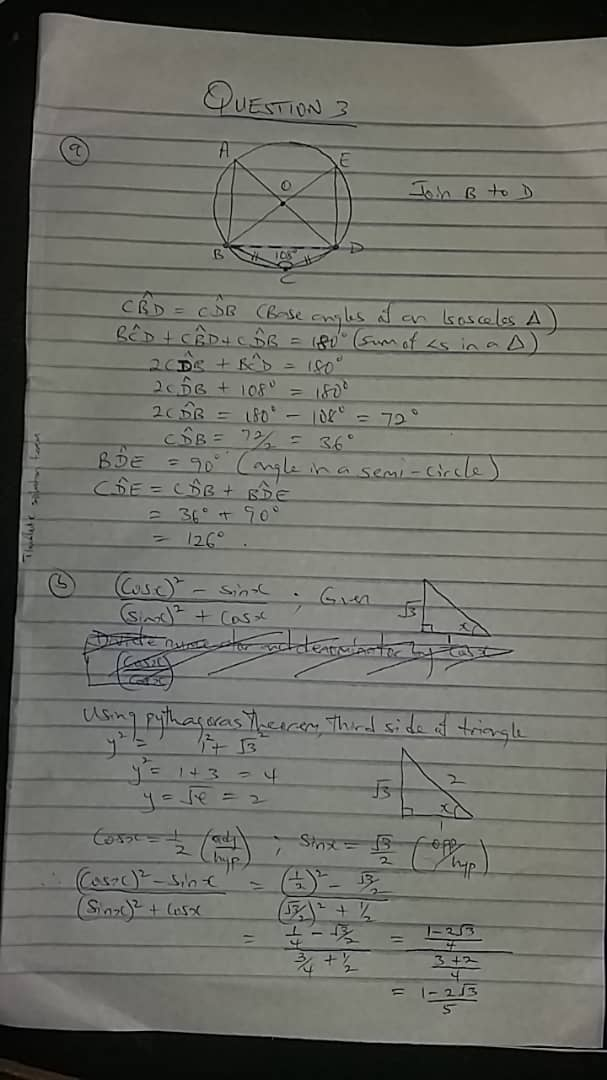View pictures in App save up to 80% data.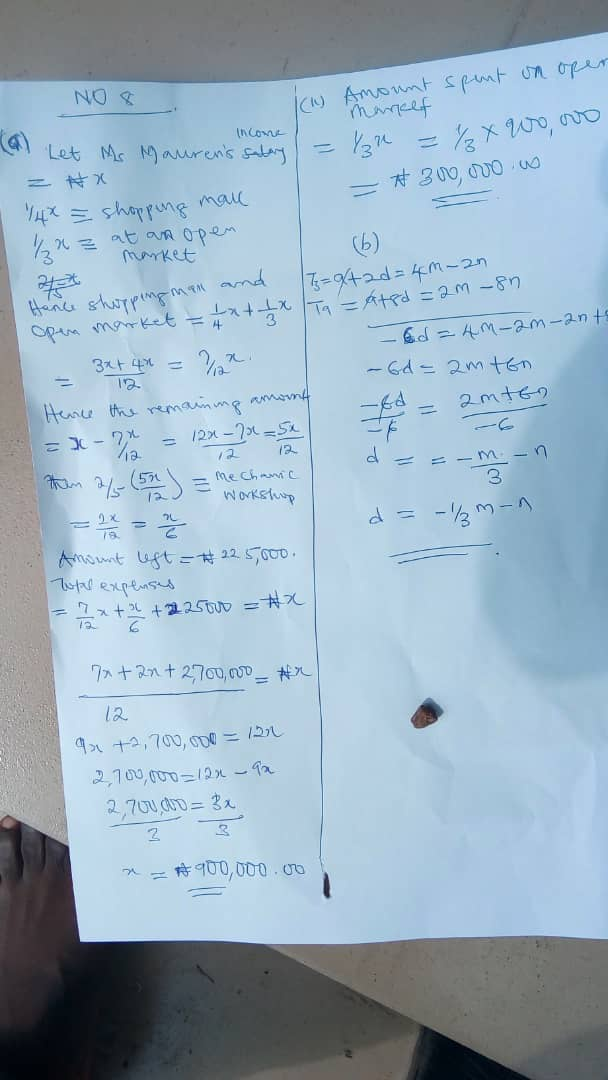View pictures in App save up to 80% data.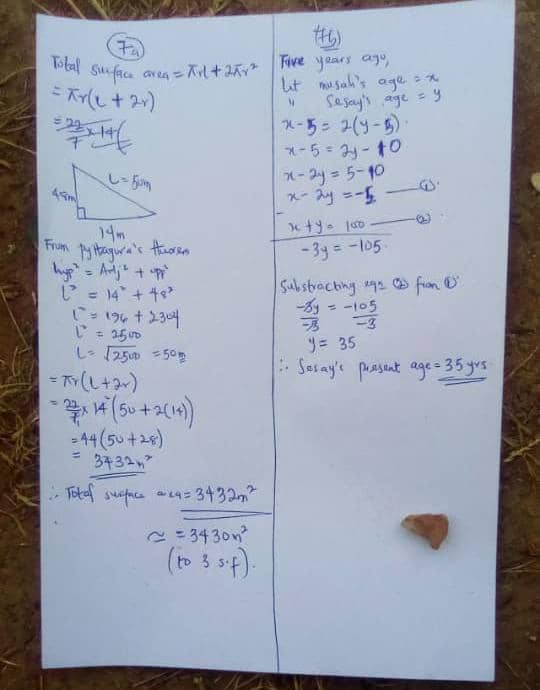View pictures in App save up to 80% data.

The objective: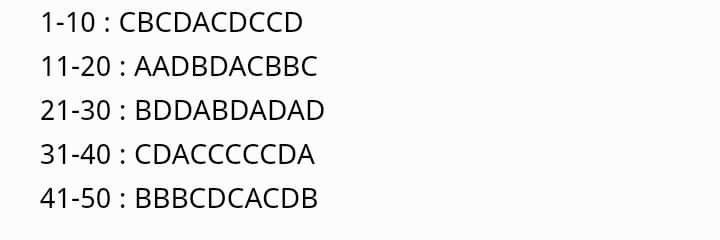View pictures in App save up to 80% data.

The above pictures are solved WAEC questions for the 17th of August 2020 and this articles was published after the examinations were concluded so the author of this post is not violating any nation laws. This article only serves as a write-up for students to see the correct answers of what they wrote.